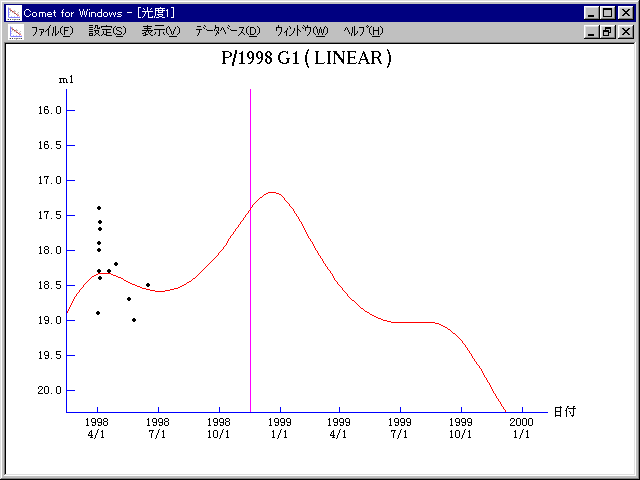# \$B%j%K%"WB@1(B

C/1998 G1 ( LINEAR )###\$B%W%m%U%#!<%k(B

 \$BH/8+F|(B 1998\$BG/(B4\$B7n(B2\$BF|(B \$BH/8+8wEY(B 18.9\$BEy(B \$BH/8+ Lincoln Laboratory Near-Earth Asteroid Research project

###\$B50F;MWAG(B

```   The following improved orbital elements by Kenji Muraoka, are
from 157 observations 1998 Apr. 2  to  June 17, perturbations
by 9 Planets, Moon and 5 minor planets were taken into account.
The mean residual is +/- 0.62 arc seconds.

Epoch  =  1998 Nov.  3.0  TT       JDT = 2451120.5
T  =  1998 Nov. 16.62892       +/- 0.00721 (m.e.) TT
Peri. =  236.32244                +/- 0.00377
Node  =  341.38353                +/- 0.00434   (2000.0)
Incl. =  109.71363                +/- 0.00142
q  =    2.1333872              +/- 0.0008238 AU
e  =    0.8235055              +/- 0.0000442
a  =   12.0875561              +/- 0.0035549 AU
n  =    0.02345289             +/- 0.00001035
P  =   42.025                  +/- 0.0185392  years
(+/- 6.77 days)
```

###\$B@1?^(B###\$B8wEYJQ2=(B

```        m1 = 14.0 + 5 log\$B&\$(B + 5.0 log r
```##### \$B50F;MWAG\$OB<2,7r<#;a\$N7W;;\$K\$h\$k\$b\$N\$G\$9!#(B \$B@1?^\$O(B StellaNavigator Ver.2.0 for Windows (\$B%"%9%H%m%"!<%D(B \$BJTCx(B / \$B%"%9%-!<=PHG6I4)(B) \$B\$G:n@.\$7\$?\$b\$N\$G\$9!#(B \$B8wEY%0%i%U\$O(BComet for Windows\$B\$G:n@.\$7\$?\$b\$N\$G\$9!#(B